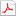검색어 입력폼

# [전기전자전파] 물리전자공학 2장 연습문제풀이

저작시기 2009.04 |등록일 2009.04.21어도비 PDF (pdf) | 29페이지 | 가격 2,000원

물리전자공학 2장 연습문제풀이

## 목차

SOLUTIONS CHAPTER 2
2.1. Of the two materials whose E-K diagrams are shown in Figure P2.1, which will have the lowest effective mass for electrons? Which will have the lowest effective mass for holes? Explain how you arrived at your conclusion.

2.2. Assume a material has an E-K diagram given by

2.3. Explain in your own words why the effective mass of an electron is different than its mass in vacuum.
중략..

## 본문내용

SOLUTIONS CHAPTER 2
2.1. Of the two materials whose E-K diagrams are shown in Figure P2.1, which will have the lowest effective mass for electrons? Which will have the lowest effective mass for holes? Explain how you arrived at your conclusion.

The effective mass is proportional to the reciprocal of the curvature of the band. The band with the greatest curvature therefore has the smallest effective mass. The E-K diagram on the right has a higher curvature in the conduction band, thus the effective mass for electrons in the conduction band is smaller than for the material on the left.
For holes, the material on the left has higher curvature and thus the effective mass for holes is smaller for the material on the left.

2.2. Assume a material has an E-K diagram given by
EK()conduction=EC+E1sin2(Ka)E(K)valence=EV−E2sin2(Ka)
Let a=0.5nm, E1=5eV, and E2=4eV.
a) Sketch the E-K diagram for the first Brillouin zone. Label the axes completely.

2.3. Explain in your own words why the effective mass of an electron is different than its mass in vacuum.
The effective mass includes the effects of the periodic potential of the crystal, so that Newtonian or classical mechanics can be used. If a given external force is applied, the effect on the electron is the sum of the external force plus all the internal forces due to the varying potential energy in the crystal. The effective mass can be used to predict the behavior of an electron in the crystal in reaction to the external force.

없음